Disclosure: This post may contain affiliate links, meaning when you click the links and make a purchase, we receive a commission.

# XIRR vs IRR – How to Calculate IRR and XIRR in Excel

The IRR (Internal Rate of Return) Function is used to calculate the internal rate of return for a series, of given periodic cash flows. These cash flows do not have to be even, though they should include the initial input investment and the subsequent values that denote the net income. The internal rate of return is utilized, in order to give an indication of the profitability of a potential investment.

Read More: Excel Protection Explained: Worksheet vs. Workbook Level Protection

The higher the internal rate of return for a particular investment, the more likely it is that the investment will be profitable. The IRR is similar to the NPV (we have already seen how to utilize the NPV Function and the XNPV Function in a previous tutorial). The IRR however, works on the premise that the NPV is 0.  The IRR input values are spaced at regular, equal intervals. The XIRR Function is used to calculate the internal rate of return for cash flows that are not periodic. The XIRR value is closely related to the XNPV function and is the internal rate of return calculated, for a corresponding XNPV of 0.

## The syntax of the IRR Excel function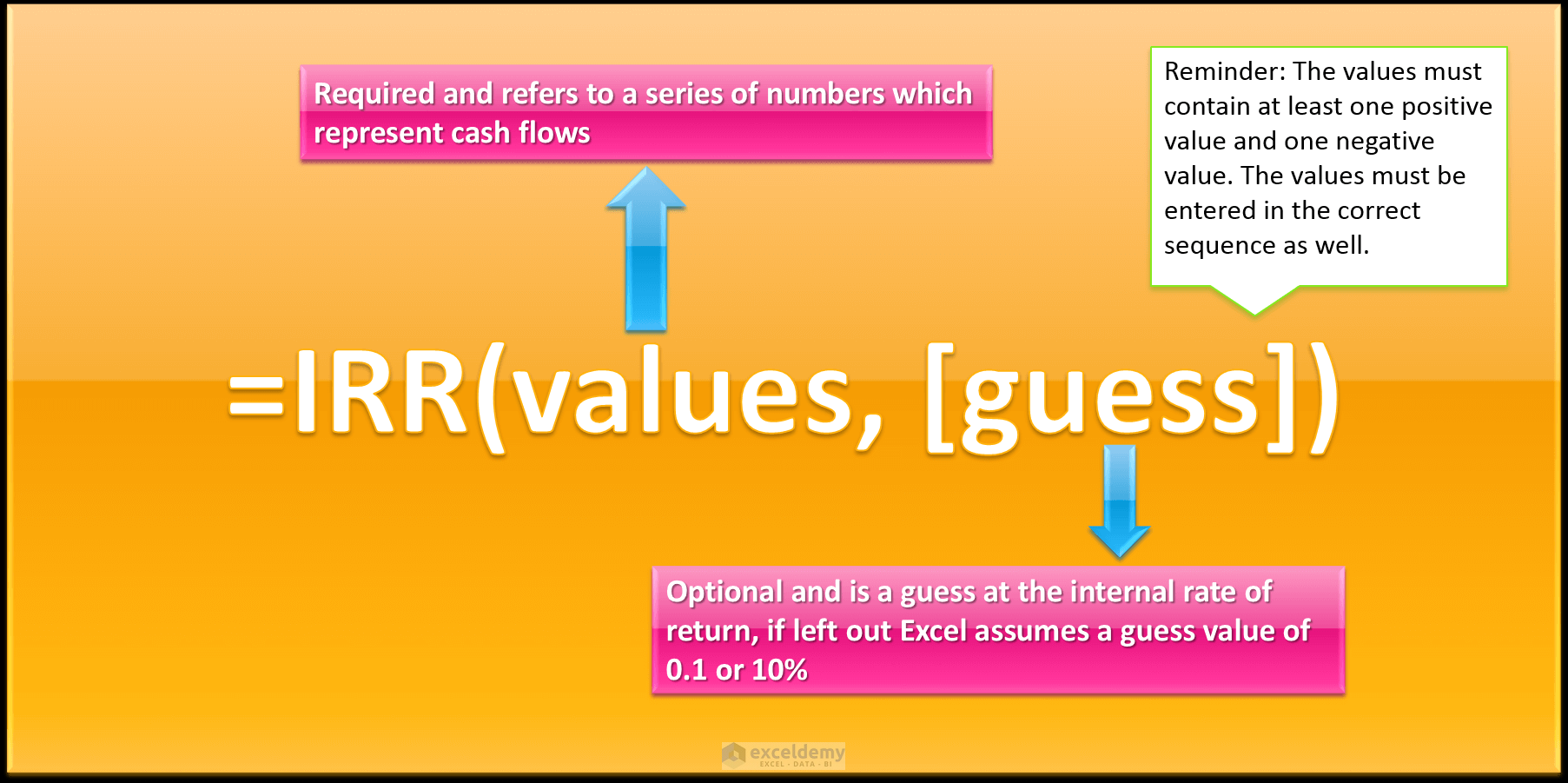## The syntax of the XIRR Excel function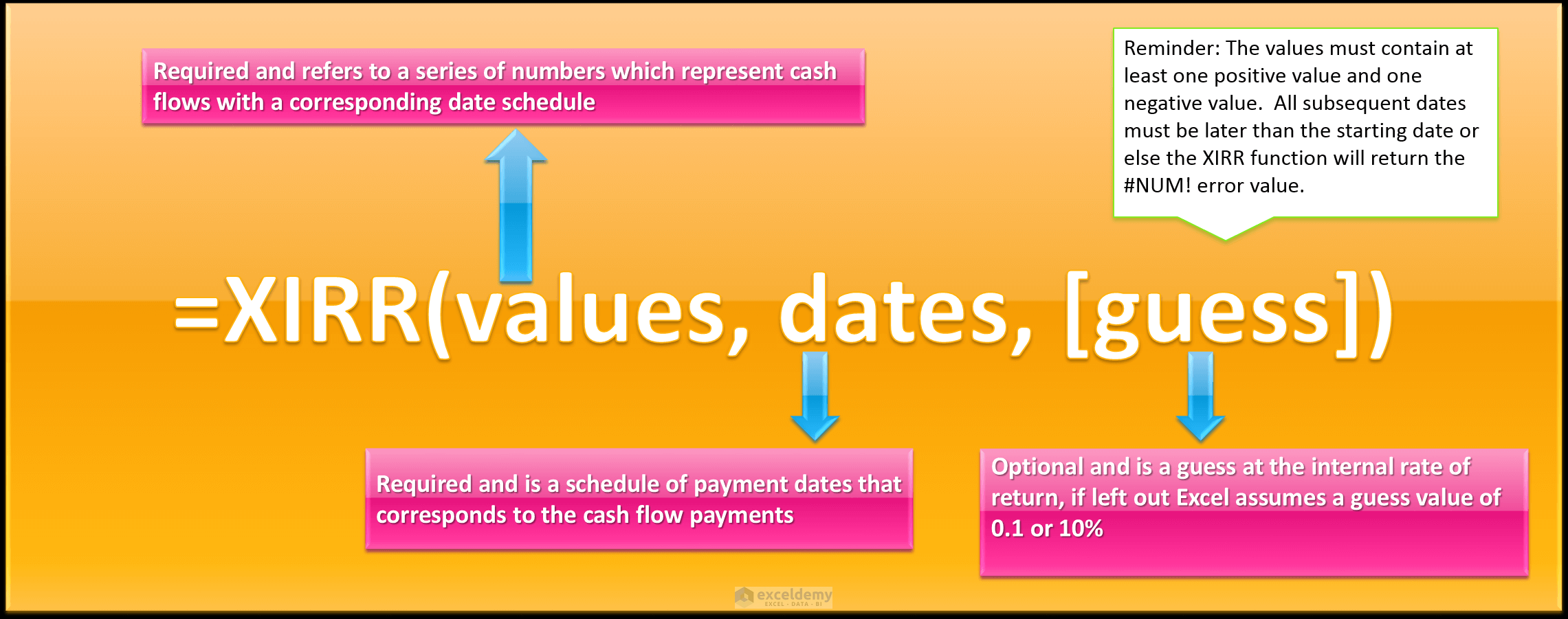So, let’s get started with a simple example to illustrate what IRR and XIRR are used for, and the main difference between them.

Read More: How to Generate Random Numbers in Excel (Ultimate Guide)

## XIRR vs IRR (Difference between IRR and XIRR)

### How to Calculate IRR in Excel

A small business owner starts a business, at a cost of \$80,000. The income for the first year is \$20,000. The income for the second year is \$22,000. The income for the third year is \$28,000 and the income for the fourth year is \$31,000. All income values refer to the net income.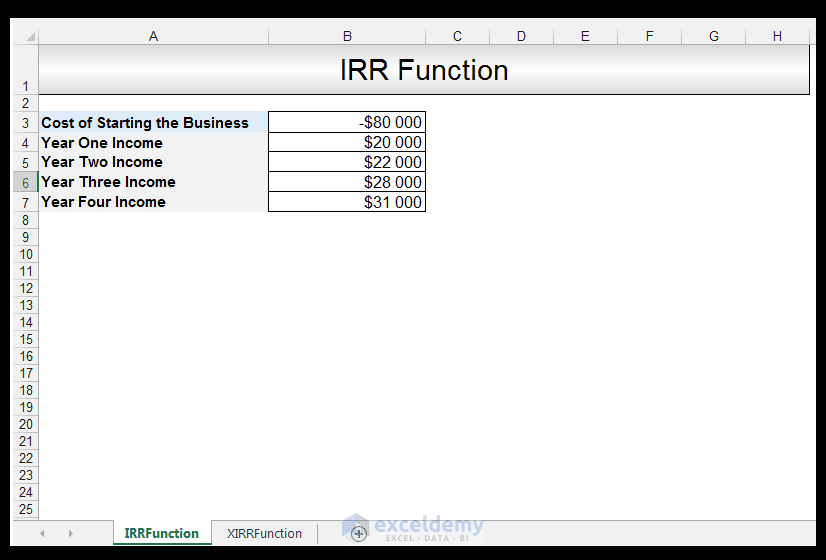1) In order to calculate the IRR, in Cell B8 we input the following formula:

=IRR (B3: B7)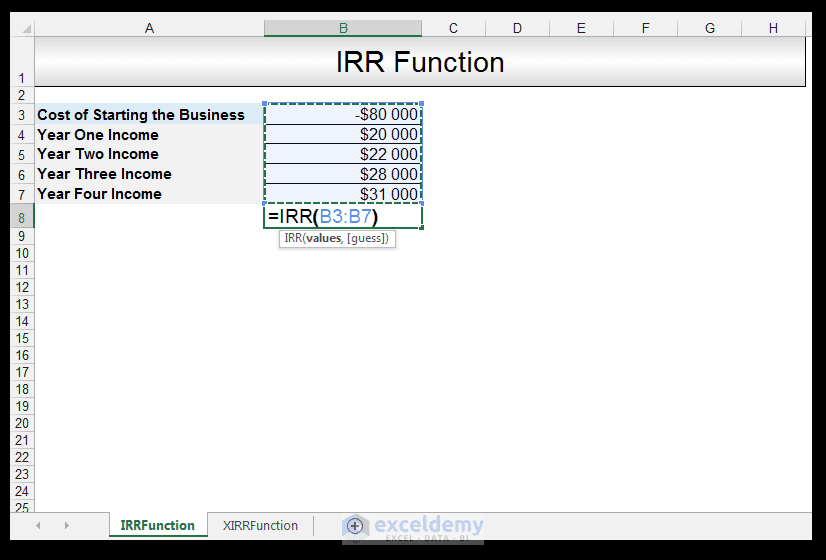2) Upon pressing CTRL-ENTER, a value of 9.2 % is returned.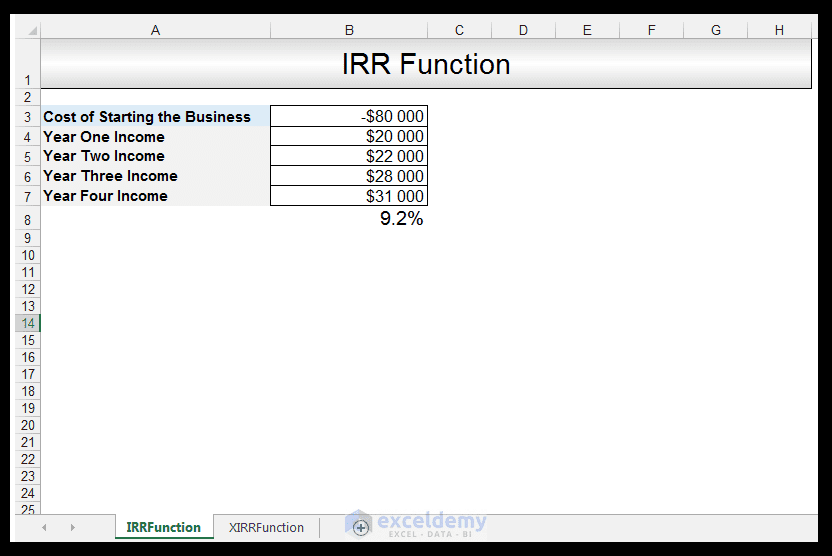The IRR of a potential project can be utilized as a comparative benchmark value, if the IRR meets the criteria of being higher than a company’s required rate of return, the company proceeds with the commencement of the project. If not, the company does not proceed with the project. The IRR can also be utilized to compare the potential profitability of multiple prospective projects, and if the cost of investment is equal for all the projects, then the project with the highest IRR would be considered to be the most attractive for investment.

### How to Calculate XIRR in Excel

A small business owner starts a construction business that has irregular cash flows, at a cost of \$85,000 on the 2 January 2015. The income for the 6 June 2015, is \$15, 000. The income for 7 October 2015 is \$10,000. The income for 10 July 2016 is \$18,000. The income for 10 January 2017 is \$19,000.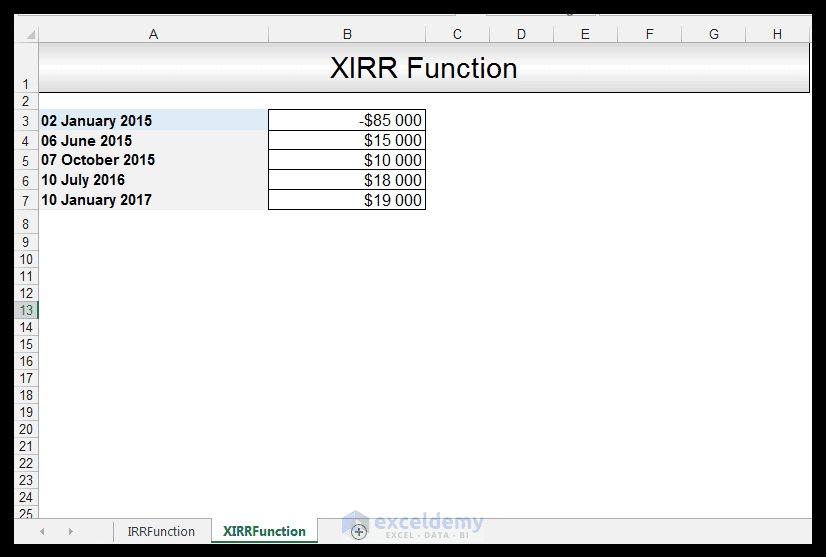1) In order to calculate the XIRR, in Cell B8 we input the following formula:

=XIRR (B3: B7, A3: A7)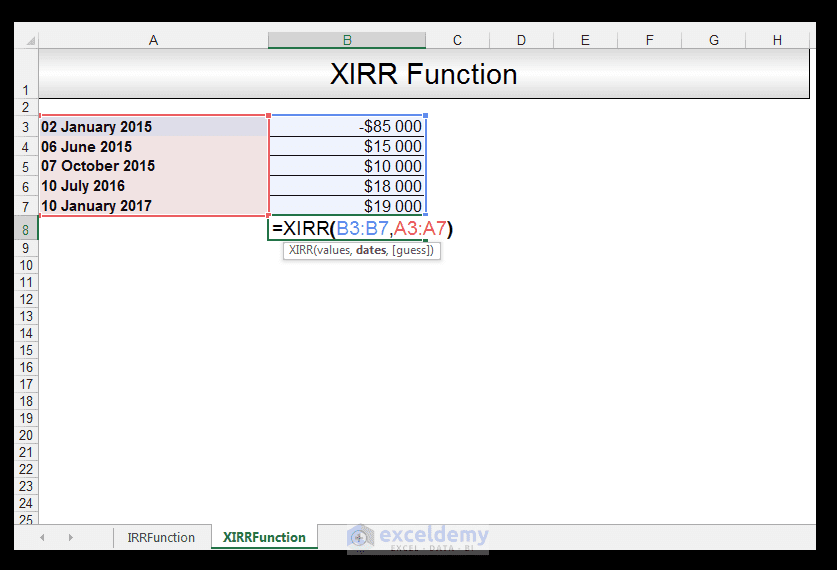2) Upon pressing CTRL-ENTER, a negative value of -21.0% is returned.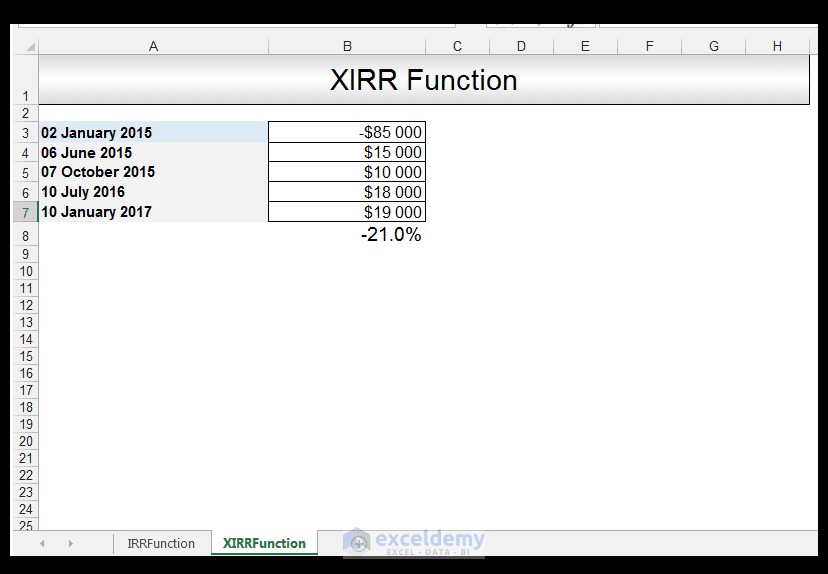This indicates a potential lack of profitability, for the time periods given.

## Conclusion

The main difference between the IRR and the XIRR Functions is that XIRR is utilized for irregular cash flows. Both give an indication of the potential profitability of a project or investment and can be utilized as a comparative benchmark. The IRR and XIRR Functions, like the NPV and XNPV, can be used as indicators of the attractiveness of a potential investment or project. The IRR and XIRR functions, however, rate attractiveness as a percentage versus an actual dollar amount (as in the case of the NPV and XNPV Functions)

Please feel free to comment and tell us what projects/investments, you use IRR and XIRR for.

NPV versus XNPV Excel Functions Tutorial

The PV, FV and PDURATION Excel Functions Tutorial

Calculating the Rate an Investment Achieves Using the RRI Function

How to Calculate Variance in Excel

IRRVersusXIRRFunction